Hostname: page-component-7d684dbfc8-dh8xm Total loading time: 0 Render date: 2023-09-27T21:11:20.918Z Has data issue: false Feature Flags: { "corePageComponentGetUserInfoFromSharedSession": true, "coreDisableEcommerce": false, "coreDisableSocialShare": false, "coreDisableEcommerceForArticlePurchase": false, "coreDisableEcommerceForBookPurchase": false, "coreDisableEcommerceForElementPurchase": false, "coreUseNewShare": true, "useRatesEcommerce": true } hasContentIssue false

# p-adic Uniformization and the Action of Galois on Certain Affine Correspondences

Published online by Cambridge University Press:  20 November 2018

## Abstract

Core share and HTML view are not possible as this article does not have html content. However, as you have access to this content, a full PDF is available via the ‘Save PDF’ action button.

Given two monic polynomials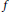$f$ and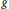$g$ with coefficients in a number field$K$, and some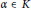$\alpha \,\in \,K$, we examine the action of the absolute Galois group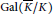$Gal\left( \bar{K}/K \right)$ on the directed graph of iterated preimages of$\alpha$ under the correspondence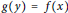$g\left( y \right)\,=\,f\left( x \right)$, assuming that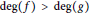$\deg \left( f \right)\,>\,\deg \left( g \right)$ and that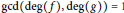$\gcd \left( \deg \left( f \right),\deg \left( g \right) \right)\,=1$. If a prime of$K$ exists at which$f$ and$g$ have integral coefficients and at which$\alpha$ is not integral, we show that this directed graph of preimages consists of finitely many$Gal\left( \bar{K}/K \right)$-orbits. We obtain this result by establishing a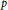$p$-adic uniformization of such correspondences, tenuously related to Böttcher’s uniformization of polynomial dynamical systems over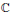$\mathbb{C}$, although the construction of a Böttcher coordinate for complex holomorphic correspondences remains unresolved.

## Keywords

Type
Research Article
Information
Canadian Mathematical Bulletin , 01 September 2018 , pp. 531 - 542